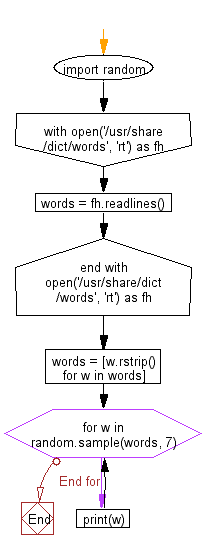﻿ Python Math: Print a random sample of words from the system dictionary - w3resource# Python Math: Print a random sample of words from the system dictionary

## Python Math: Exercise-54 with Solution

Write a Python program to print a random sample of words from the system dictionary.

Sample Solution:-

Python Code:

``````import random
with open('/usr/share/dict/words', 'rt') as fh:
words = [w.rstrip() for w in words]
for w in random.sample(words, 7):
print(w)
```
```

Sample Output:

```philatelist's
thieve
sparrows
Loretta's
hostiles
naughty
afflicts
```

Flowchart:Python Code Editor:

Have another way to solve this solution? Contribute your code (and comments) through Disqus.

What is the difficulty level of this exercise?

Test your Python skills with w3resource's quiz

﻿

## Python: Tips of the Day

Python: Get the Key Whose Value Is Maximal in a Dictionary

```>>> model_scores = {'model_a': 100, 'model_z': 198, 'model_t': 150}
>>> # workaround
>>> keys, values = list(model_scores.keys()), list(model_scores.values())
>>> keys[values.index(max(values))]
'model_z'
>>> # one-line
>>> max(model_scores, key=model_scores.get)
'model_z'
```